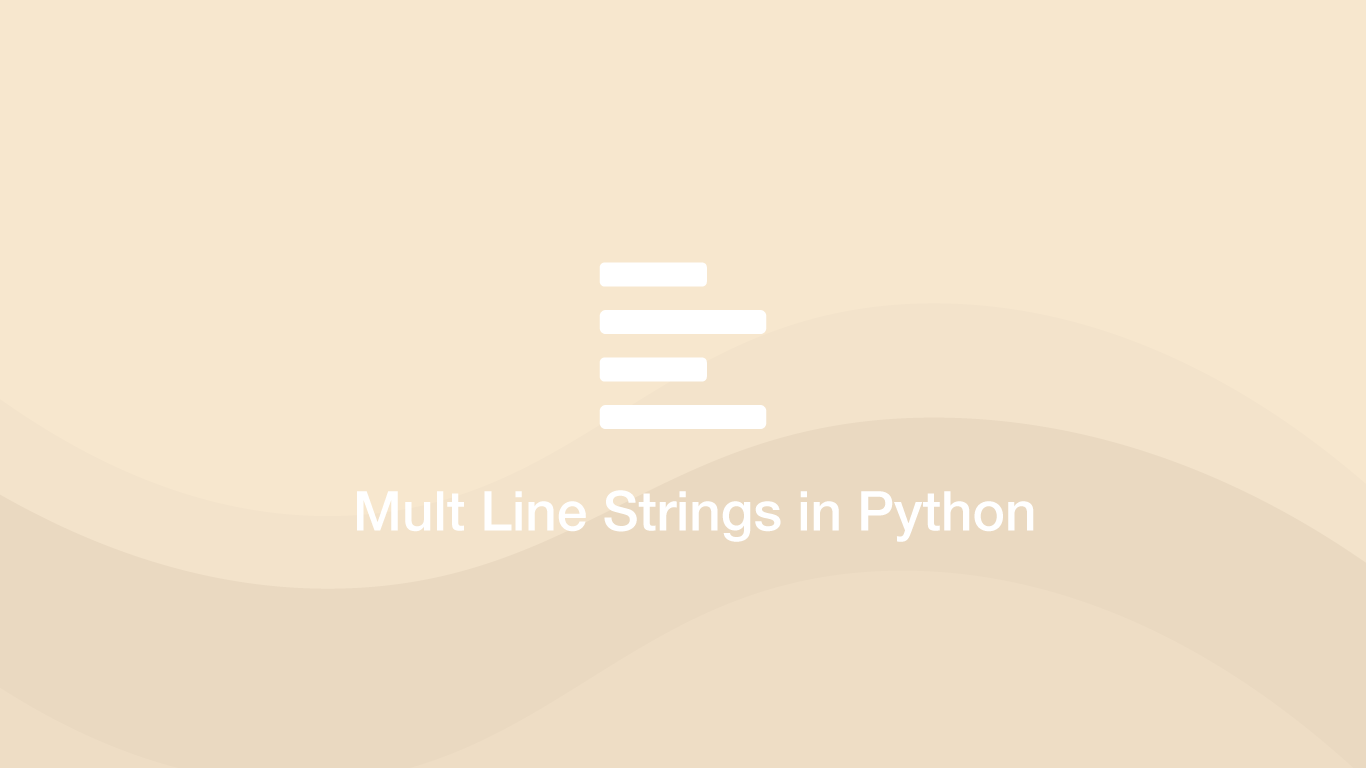# How to Create Multi-Line Strings in PythonThere are several ways to create multi-line strings in Python. Let's have a look at some of the different approaches we can take to solve this problem.

## Using Three Double Quotes

The easiest way to create a multiline string is to wrap the string in opening and closing `"""` (three double quotes) and assign the value to a variable. The new lines represented in the program will also be how the string is printed.

``````string = """hello this is a line
and this is a line
and another line
"""

print(string)
``````
``````hello this is a line
and this is a line
and another line
``````

## Using Three Single Quotes

The string can also be wrapped in `'''` (three single quotes).

``````string = '''hello this is a line
and this is a line
and another line
'''

print(string)
``````
``````hello this is a line
and this is a line
and another line
``````

## Using Parenthesis and Quotes

A single line string can be built on multiple lines in the program by wrapping it in `()` (parenthesis) and wrapping each line in `""` (double quotes) or `''` (single quotes) and storing the string in a variable.

``````string = ("hello this is a line"
"and this is a line"
"and another line")

print(string)
``````
``````hello this is a lineand this is a lineand another line
``````

note - as you can see from the output no extra spaces or newlines are included.

## Create an Array and Convert it to a String

Another approach is to create an array of strings and then join them back together using a `\n` (newline) as the glue. We can do this using the .join() method.

``````array = ["hello this is a line", "and this is a line", "and another line"]

glue = '\n'

string = glue.join(array)

print(string)
``````
``````hello this is a line
and this is a line
and another line
``````

## Conclusion

You now know how to create multi-line strings in Python in several different ways depending on your particular situation.